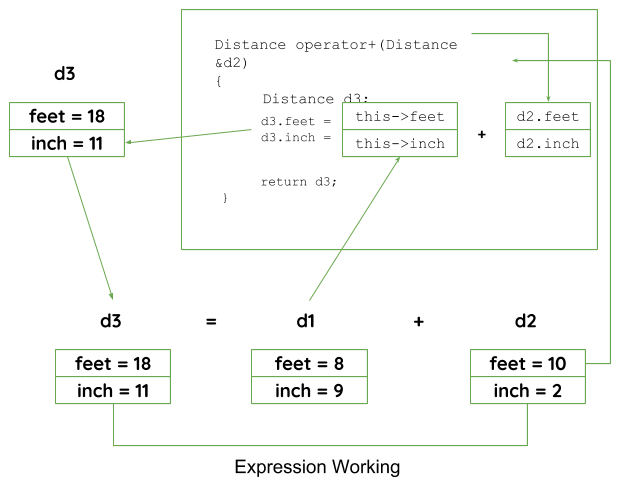• Difficulty Level : Medium
• Last Updated : 17 May, 2021

C++ provides a special function to change the current functionality of some operators within its class which is often called as operator overloading. Operator Overloading is the method by which we can change the function of some specific operators to do some different task.

This can be done by declaring the function, its syntax is,

```Return_Type classname :: operator op(Argument list)
{
Function Body
}```

In the above syntax Return_Type is value type to be returned to another object, operator op is the function where the operator is a keyword and op is the operator to be overloaded.
Operator function must be either non-static (member function) or friend function.

Below are some criteria/rules to define the operator function:

• In case of a non-static function, the binary operator should have only one argument and unary should not have an argument.
• In the case of a friend function, the binary operator should have only two argument and unary should have only one argument.
• All the class member object should be public if operator overloading is implemented.
• Operators that cannot be overloaded are . .* :: ?:
• Operator cannot be used to overload when declaring that function as friend function = () [] ->.

1. Overloading Unary Operator: Let us consider to overload (-) unary operator. In unary operator function, no arguments should be passed. It works only with one class objects. It is a overloading of an operator operating on a single operand.
Example:
Assume that class Distance takes two member object i.e. feet and inches, create a function by which Distance object should decrement the value of feet and inches by 1 (having single operand of Distance Type).

## CPP

 `// C++ program to show unary operator overloading``#include ` `using` `namespace` `std;` `class` `Distance {``public``:` `    ``// Member Object``    ``int` `feet, inch;` `    ``// Constructor to initialize the object's value``    ``Distance(``int` `f, ``int` `i)``    ``{``        ``this``->feet = f;``        ``this``->inch = i;``    ``}` `    ``// Overloading(-) operator to perform decrement``    ``// operation of Distance object``    ``void` `operator-()``    ``{``        ``feet--;``        ``inch--;``        ``cout << ``"\nFeet & Inches(Decrement): "` `<< feet << ``"'"` `<< inch;``    ``}``};` `// Driver Code``int` `main()``{``    ``// Declare and Initialize the constructor``    ``Distance d1(8, 9);` `    ``// Use (-) unary operator by single operand``    ``-d1;``    ``return` `0;``}`

1.
Output:

`Feet & Inches(Decrement): 7'8`

1. In the above program, it shows that no argument is passed and no return_type value is returned, because unary operator works on a single operand. (-) operator change the functionality to its member function.
Note: d2 = -d1 will not work, because operator-() does not return any value.
Let’s take the same example of class Distance, but this time, add two distance objects.

## CPP

 `// C++ program to show binary operator overloading``#include ` `using` `namespace` `std;` `class` `Distance {``public``:``    ``// Member Object``    ``int` `feet, inch;``    ``// No Parameter Constructor``    ``Distance()``    ``{``        ``this``->feet = 0;``        ``this``->inch = 0;``    ``}` `    ``// Constructor to initialize the object's value``    ``// Parameterized Constructor``    ``Distance(``int` `f, ``int` `i)``    ``{``        ``this``->feet = f;``        ``this``->inch = i;``    ``}` `    ``// Overloading (+) operator to perform addition of``    ``// two distance object``    ``Distance operator+(Distance& d2) ``// Call by reference``    ``{``        ``// Create an object to return``        ``Distance d3;` `        ``// Perform addition of feet and inches``        ``d3.feet = ``this``->feet + d2.feet;``        ``d3.inch = ``this``->inch + d2.inch;` `        ``// Return the resulting object``        ``return` `d3;``    ``}``};` `// Driver Code``int` `main()``{``    ``// Declaring and Initializing first object``    ``Distance d1(8, 9);` `    ``// Declaring and Initializing second object``    ``Distance d2(10, 2);` `    ``// Declaring third object``    ``Distance d3;` `    ``// Use overloaded operator``    ``d3 = d1 + d2;` `    ``// Display the result``    ``cout << ``"\nTotal Feet & Inches: "` `<< d3.feet << ``"'"` `<< d3.inch;``    ``return` `0;``}`

1.
Output:

`Total Feet & Inches: 18'11`

1. Here in the above program,
See Line no. 26, Distance operator+(Distance &d2), here return type of function is distance and it uses call by references to pass an argument.
See Line no. 49, d3 = d1 + d2; here, d1 calls the operator function of its class object and takes d2 as a parameter, by which operator function return object and the result will reflect in the d3 object.
Pictorial View of working of Binary Operator:1. Overloading Binary Operator using a Friend function: In this approach, the operator overloading function must precede with friend keyword, and declare a function class scope. Keeping in mind, friend operator function takes two parameters in a binary operator, varies one parameter in a unary operator. All the working and implementation would same as binary operator function except this function will be implemented outside of the class scope.
Let’s take the same example using the friend function.

## CPP

 `// C++ program to show binary operator overloading``#include ` `using` `namespace` `std;` `class` `Distance {``public``:` `    ``// Member Object``    ``int` `feet, inch;` `    ``// No Parameter Constructor``    ``Distance()``    ``{``        ``this``->feet = 0;``        ``this``->inch = 0;``    ``}` `    ``// Constructor to initialize the object's value``    ``// Parameterized Constructor``    ``Distance(``int` `f, ``int` `i)``    ``{``        ``this``->feet = f;``        ``this``->inch = i;``    ``}` `    ``// Declaring friend function using friend keyword``    ``friend` `Distance operator+(Distance&, Distance&);``};` `// Implementing friend function with two parameters``Distance operator+(Distance& d1, Distance& d2) ``// Call by reference``{``    ``// Create an object to return``    ``Distance d3;` `    ``// Perform addition of feet and inches``    ``d3.feet = d1.feet + d2.feet;``    ``d3.inch = d1.inch + d2.inch;` `    ``// Return the resulting object``    ``return` `d3;``}` `// Driver Code``int` `main()``{``    ``// Declaring and Initializing first object``    ``Distance d1(8, 9);` `    ``// Declaring and Initializing second object``    ``Distance d2(10, 2);` `    ``// Declaring third object``    ``Distance d3;` `    ``// Use overloaded operator``    ``d3 = d1 + d2;` `    ``// Display the result``    ``cout << ``"\nTotal Feet & Inches: "` `<< d3.feet << ``"'"` `<< d3.inch;``    ``return` `0;``}`

1.
Output:

`Total Feet & Inches: 18'11`

1. Here in the above program, operator function is implemented outside of class scope by declaring that function as the friend function.

In these ways, an operator can be overloaded to perform certain tasks by changing the functionality of operators.

My Personal Notes arrow_drop_up